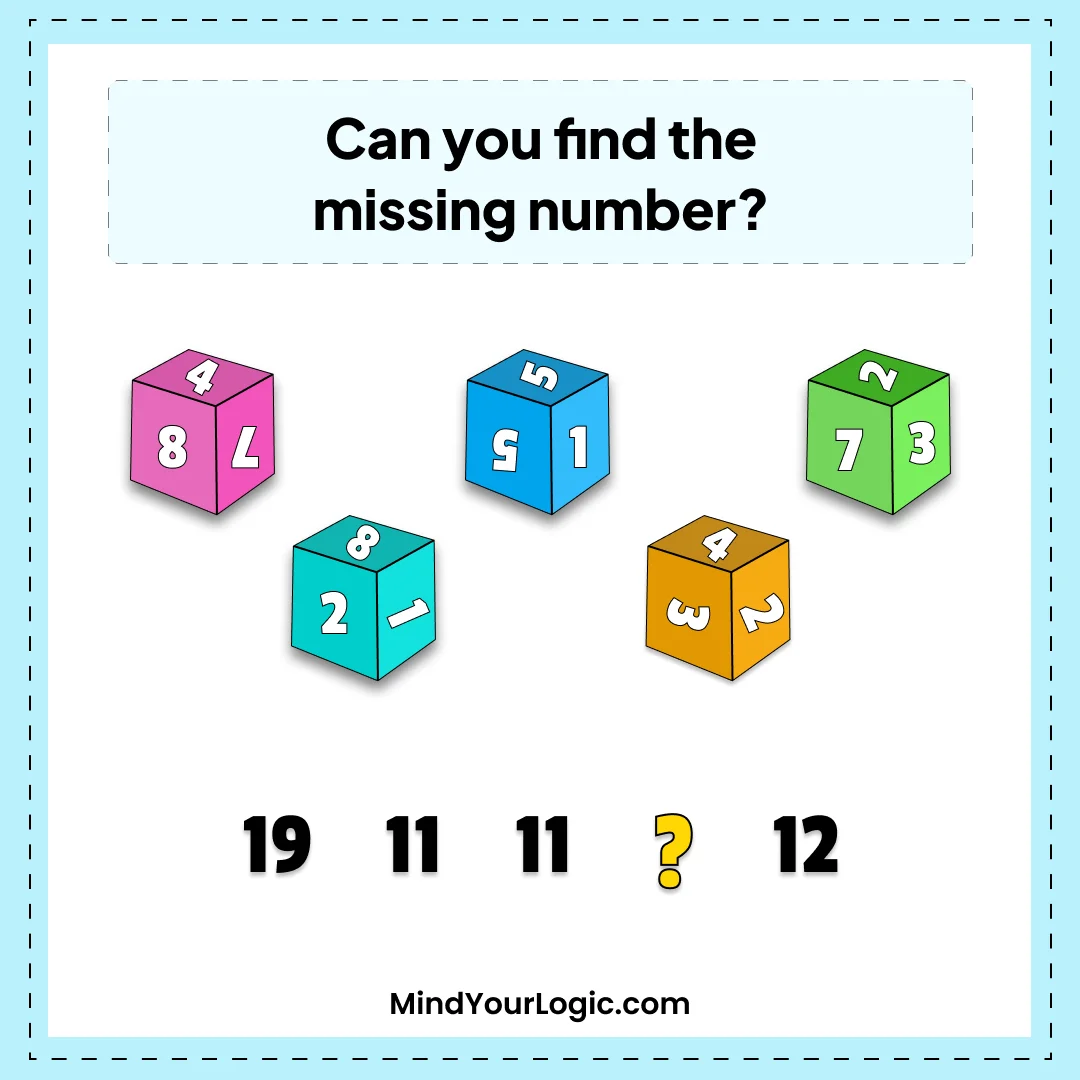# Missing Number - Math Riddle

###### 78.Math Riddles
`Can you find the missing number?`• A.9
• B.6
• C.10
• D.7
• ```Explanation :

The number that should replace the question mark is 9.
Why?
The sum of the three numbers on each die equals the number written below it.
For the first die, for example: 8 + 4 + 7 = 19;
for the second: 8 + 2 + 1 = 11.
Following this pattern would explain why the third die is also 11 (5 + 5 + 1)
and why the mysterious question mark should be 9 (4 + 3 + 2).```